American Journal of Modern Physics
Volume 5, Issue 4-1, July 2016, Pages: 1-5

Cosmology of Einstein’s NOW

Amrit Sorli1, Vlad Koroli2, Andrei Nistreanu2, Davide Fiscaletti3

1Foundations of Physics Institute, Idrija, Slovenia

2Institute of Applied Physics, Academy of Sciences of Moldova, Kishinev, Moldova

3SpaceLife Institute, San Lorenzo in Campo (PU), Italy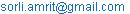(A. Sorli)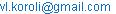(V. Koroli)(A. Nistreanu)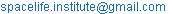(D. Fiscaletti)

Amrit Sorli, Vlad Koroli, Andrei Nistreanu, Davide Fiscaletti. Cosmology of Einstein’s NOW. American Journal of Modern Physics. Special Issue: Insufficiency of Big Bang Cosmology. Vol. 5, No. 4-1, 2016, pp. 1-5. doi: 10.11648/j.ajmp.s.2016050401.11

Abstract: Bijective epistemology results confirm models of "space-time" and "empty" space have no counterpart in physical universe. Time is a numerical order of material changes, i.e. motion in universal space where time is not its 4th dimension. NASA results confirm universal space is "flat", means it corresponds to the Euclidean geometry. Riemann finite geometry seems not to be an adequate model of universal space. The only possible alternative is infinite Euclidean universal space which has origin in quantum vacuum where is always NOW. Universe does not run in time, on the contrary: time is a numerical order of material changes, i.e. motion which run in quantum vacuum.

Keywords: Cosmology, Time, Quantum Vacuum, Bijective Epistemology, Gravitational Constant G

1. Introduction

Our recent research on bijective epistemology confirms time is not a 4th dimension of space and space-time model has no a counterpart in physical universe. Further on bijective epistemology denies that an "empty space" deprived of physical properties could be a medium in which run physical changes. Term "empty space" has no counterpart in physical universe, exists only as a mathematical model . These results require that universal space has some more fundamental origin; in this paper is proposed origin of universal space is quantum vacuum of Planck metrics which has variable energy density. A given particle or massive body diminishes energy density of quantum vacuum respectively to the amount of its energy. Results of our recent research confirm curvature of space-time model in General Relativity is a description of energy density of quantum vacuum . Our model is supported by the results of NASA research which confirm concrete universal space is not curved; it corresponds to the Euclidean geometry . Considering that universal space is flat model of "space curvature" has counterpart in some ontologically deeper physical reality which is variable energy density of quantum vacuum.

In the theory of infinite numbers is known that if we say that cardinal number of natural numbers is equal to the cardinal number of real numbers, we do not enter in contradiction. If we say that cardinal number of real numbers is bigger than cardinal numbers of natural numbers, we also do not enter in contradiction. This confirms that "infinity" is not a metric term. Infinite distance plus 1000 km is still infinite distance. Considering that Euclidean space corresponds to the geometrical form of universal space we will have difficulties to approach universe as a close system which has finite amount of matter and finite diameter. It is more reasonable to study laws of observable universe which homogeneity assure that the same laws are valid in entire infinite universe.

2. Cosmological Model "Universe in Dynamic Equilibrium" – UDE

In quantum vacuum which is the fundamental arena of the universe time has merely a mathematical existence. Past, present and future are not 4th dimension of space, they have only a mathematical existence . Universe exists in what Albert Einstein use to call NOW: "…there is something essential about the NOW which is just outside the realm of science. People like us, who believe in physics, know that the distinction between the past, present and future is only a stubbornly persistent illusion" . Common picture of the universe expanding in time is not appropriate, see figure below: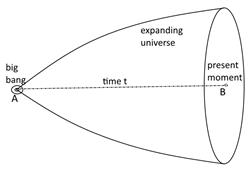Fig. 1. Imagine of Big bang cosmology.

Universe exists in quantum vacuum (from now on "dynamic quantum vacuum" – DQV which is NOW. Universe is timeless in a sense that time is not a physical dimension in which universe exists. This view is also confirmed by the research of Kurt Gödel. By 1949, Gödel had produced a remarkable proof: "In any universe described by the Theory of Relativity, time cannot exist" .

Model of the universe where time is only a mathematical parameter of change, i.e. motion requires the re-reading of some experimental data. Considering that universe exists in physical time the interpretation that cosmic microwave background radiation (CMB) has a source 380000 years after big bang  makes sense because time t is a physical dimension which relates big bang (on the Figure 1 point A) with present moment (on the Figure 1 point B) in which we measure CMB; with other words time is a transmitter of CMB. Considering that universe exists in DQV which is NOW, CMB cannot have its source in some hypothetical physical past and time cannot be transmitter of CMB.

The source of CMB is present in actual universe that we observe. In this perspective also BICEP2 model of gravitational waves as ripples of space-time which have origin in big bang is questionable. Gravitational waves (if they exist) should have origin in DQV which is NOW. In our model gravity is a result of fundamental symmetry between given particle or material object and diminished energy density of DQV. Our model does not predict existence of hypothetical graviton and also does not predict existence of gravitational waves. Mass and gravity have both origin in diminished energy density of quantum vacuum caused by the presence of a given particle or massive body .

NASA results confirm with 0.4% margin of error universal space is "flat" . This means observable universal space has a form of Euclidean space. In General Relativity (GR) universal space is curved. Between NASA results and curved space in GR seems to be a discrepancy. In our model curvature of space in GR has origin in a variable energy density of dynamic quantum vacuum (further on "DQV"). There is no discrepancy between NASA results and model of curved space in GR.

Stability of elementary particles requires a certain energy density of DQV. In the centre of black holes energy density of DQV is at the minimum and reaches below required energy density which is giving stability to elementary particles. In singularities elementary are not stable anymore and disintegrates back into fundamental primordial energy of DQV.

In intergalactic areas energy density of DQV is at the maximum. In intergalactic areas energy of DQV is continuously transforming in cosmic rays and this further in elementary particles . This circulation of energy "matter – DQV– matter" is in a permanent dynamic equilibrium.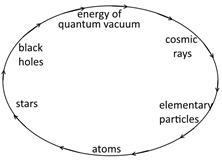Fig. 2. Permanent circulation of energy in the universe.

In black holes singularities "old matter" is transformed into "fresh" fundamental primordial energy of DQV which is not created and cannot be destroyed. Increasing of entropy of matter that we observe in the universe does not increase the entropy of entire universe which as the whole has no entropy. Black holes are rejuvenating universe which is ageless. According to our cosmological model Universe in Dynamic Equilibrium - (UDE), universe is a non-created system.

The question of "the beginning" and "the end" of the universe seems not to be right one. In the universe galaxies, stars and planets appear and disappear; universe itself is eternal. This model of the universe has much more epistemological stability than all other models which predict beginning of the universe. Idea of the beginning comes out of human imagination that universe exists in some linear time which is physical reality. Our research confirms time we measure with clocks has only a mathematical existence; it is a numerical order of changes running in quantum vacuum. Past, present and future belong to the psychological time in which we experience flow of changes running in quantum vacuum where is always NOW .

3. Calculations of Density of DQV and Value of Gravitational Constant G

In intergalactic space density of DQV has a value of Planck density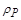:(1)In the centre of black hole with the mass M of the Sun and radius r of 3000 meters density of DQV is following: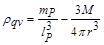(2)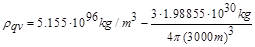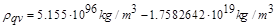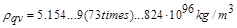The difference between density of quantum vacuum in interstellar space and in the centre of the black hole is infinitesimal; however variable density of quantum vacuum is the driving force of permanent energy circulation in the universe which is described in a chapter 2.

Value of gravitational constant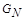in intergalactic space far away from stellar objects can be expressed with Planck units:(3)

where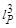is Planck volume,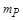is Planck mass and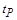is Planck time. Formalism (3) can also be written: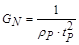(4)

whereis Planck density. In interstellar space the value of gravitational constantis following: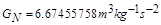Calculations of the impact of variable density of quantum vacuum on the value of gravitational constant in the centre of black hole are confirming that value of gravitational constant remains unchanged comparing to the value of gravitational constant in intergalactic space.

Below is calculation of gravitational constantvalue in the centre of the black hole with mass of the Sun according to the formalism (5) which is valid for all stellar objects: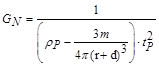(5)

where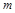is the mass of the stellar object,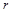is the radius ,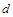is distance from the centre of stellar object. When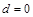one get value of gravitational constant in the centre of stellar object. Whenone get value of gravitational constant on the surface of the stellar object. When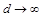one gets value of gravitational constantin intergalactic space far away from stellar objects.This calculation confirms that in cosmology we can consider value of gravitational constantis not changing. Presence of stellar objects diminishes density and energy density of quantum vacuum; however value of gravitational constantremains unchanged.

Recent publication of Caligiuri is suggesting that value of gravitational constant is changing with changing of density of quantum vacuum and is different in the centre of the Earth than on the Earth surface . According to the calculations in this paper planet Earth mass which is much smaller than black hole mass cannot influence value of gravitational constant. Our calculations confirm that presence of massive objects in a given area of quantum vacuum do not influence value of gravitational constant.

Gravitational constanthas been measured by several groups in different places on the globe and the values are variable from 0,1% to 0,7% . Results of last 30 years of measurement of gravitational constant show that there is some measurement error or either some "strange" influence is affecting most ofmeasurement: "The situation is disturbing — clearly either some strange influence is affecting most G measurements or, probably more likely, measurements of G since 1980 have unrecognized large systematic errors. The need for new measurements is clear". Anderson and others speculate in their article that variability ofcould not have origin in changing motions in the Earth's core, or perhaps some other geophysical process: "Least unlikely, perhaps, are currents in the Earth’s fluid core that change both its moment of inertia (affecting LOD) and the circumstances in which the Earth-based experiments measure G" . Our calculation ofvalue in the centre of black hole confirms their speculation. Motions of Earth’s core masses are much smaller than amount of masses in the black hole and cannot influence value of.

Least unlikely, perhaps, as Earth is not perfectly round one could suppose that value of gravitational constantdepends on the distance from the Earth centre. Here are calculations ofon the pole and on the equator based on formalism (5):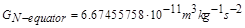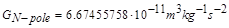Following calculation is about the value ofin the centre of the Earth based on formalism (5):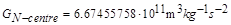Calculations confirm there is no difference between the values ofon the equator, pole and in the centre.

Following calculation is about value of20000 km above the Earth surface according to the formalism (5):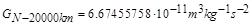Value of20000 km above the Earth surface remains unchanged.

Distance between Earth and Moon at perihelion is 363104 km, at aphelion distance is 405696 km. Mass of the Moon is 7.34767309 × 1022 kilograms, radius of the Moon is 1737.1 km. When Moon is at perihelion value foron the Earth has according to formalism (5) following value: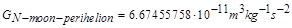When Moon is at aphelion value foron the Earth has according to formalism (5) following value:We can see that the Moon motion has no influence on variability ofon the Earth.

Next calculation is about the Sun motion influence on variability of gravitational constanton the Earth on the basis of equation (5). Distance between Earth and Sun at perihelion is 147098074 km, at aphelion distance is 152,097,701 km. Mass of the Sun is 1.98855 ×1030 kilograms, radius of the Sun is 696342 km.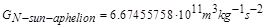Calculations confirm that motion Sun does not influence value of gravitational constanton the Earth surface.

Measurements ofin last 30 years have been done at different times and on different places. We are proposing an experiment wherewill be measured at the same time on different places on the Earth. According to our model values ofshould be the same on all different places. In our model the only factor which influences value of gravitational constant is density of dynamic quantum vacuum (DQV). Calculations confirm that also in a centre of black hole where we have lowest density of quantum vacuum, gravitational constant remains unchanged. If we will get different values forin proposed experiment which we plan in 2016, we can than speculate that there is (beside density of dynamic quantum vacuum) some unknown factor which influences variability of.

4. Epistemological Insufficiency of Inflation in Big bang Cosmology

According to the Big bang cosmological model universe has started from the infinitesimally small amount of energy which has an enormous density. Then in the first period matter and space have been created continuously. This period is called "Inflation". From where energy of matter and energy of space has been appearing Big Bang model does not explain. Stephen Hawking explains inflation period in his book "The brief History of time" with mathematics. He says that energy of matteris positive and energy of gravity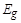(which is energy of space) is negative. The sum of these two energies in the universe is always zero :(6)

Hawking suggests that in inflation phase this two energies are multiplying similarly as we can multiply natural numbers and their sum remains always zero: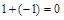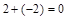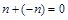(7)

Validity of equation (7) does not mean that energy of matter and gravitational energy can multiply out of nothing. This explanation of inflation phase is against first law of thermodynamics has no common sense. According to bijective epistemology mathematics can describe universe and cannot explain it. Einstein use to say: "As far as the laws of mathematics refer to reality, they are not certain; and as far as they are certain, they do not refer to reality" .

Idea that universe is expanding makes sense only if we propose universe is finite. Only a finite system can expand. NASA results confirm universal space correspond Euclidean geometry and is infinite . Infinite system cannot expand and cannot contact. Expansion of observable universe is questionable because "red shift" can also be interpreted as a consequence of light pulling from the strong gravity . This so called "gravitational red shift" is a basis for "tired light" hypothesis of Swiss astronomer Fritz Zwicky. He proposed that the reddening effect was not due to motions of the galaxy, but to an unknown phenomenon that caused photons to lose energy as they travelled through space. He considered the most likely candidate process to be a drag effect in which photons transfer momentum to surrounding masses through gravitational interactions; and proposed that an attempt be made to put this effect on a sound theoretical footing with general relativity. He also considered and rejected explanations involving interactions with free electrons, or the expansion of space . Considering that red shift has gravitational origin, expansion of observable universe is not certain any more.

5. Conclusions

Bijective epistemology based on bijective function of set theory fulfils Einstein’s vision about completeness of a given scientific model which requires that each element of a model has exactly one counterpart in the universe. Application of bijective epistemology in cosmology suggests that universe is a non-created system in a permanent dynamic equilibrium. Man is born and he dies. He thinks the same about universe. Our paper shows this "birth-death" approach is not applicable on the universe. Universe is a non created system in a permanent dynamic equilibrium, where time is merely a mathematical parameter of material changes.

References

1. Fiscaletti, D. and Sorli, A.: "Bijective Epistemology and Space-Time", Foundations of Science, Springer, Volume 20, Issue 4, pp 387-398 (2015).
2. Fiscaletti, D. and Sorli, A.: "Space-time curvature of general relativity and energy density of a three-dimensional quantum vacuum", Annales UMCS Sectio AAA: PhysicaLXIX, 55-81 (2014).
3. NASA, http://map.gsfc.nasa.gov/universe/uni_shape.html (2013).
4. Fiscaletti, D. and Sorli, A.: "Perspectives of the Numerical Order of Material Changes in Timeless Approaches in Physics", Foundations of Physics 45, 2, 105-133 (2015).
5. A. Sorli, The Physics of NOW, Amazon, (2014).
6. A. Sorli, D. Fiscaletti, T. Gregl: New Insights into Gödel's Universe without Time, Volume 26, Num 1, pp. 113-115, Physics Essays (2013).
7. Wilson, R. W.; Penzias, A. A."Isotropy of Cosmic Background Radiation at 4080 Megahertz". Science 156 (3778): 1100–1101. doi:10.1126/science.156.3778.1100. (1967).
8. Luigi Maxmilian Caligiuri, Amrit Sorli, Gravity Originates from Variable Energy Density of Quantum Vacuum, American Journal of Modern Physics. Vol. 3, No. 3, 2014, pp. 118-128. doi:10.11648/j.ajmp.20140303.11.
9. M. W. Friedlander, A Thin Cosmic Rain: Particles from Outer Space, Harvard University Press, Harvard. (2002).
10. Luigi Maxmilian Caligiuri, Gravitational "Constant" G as a Function of Quantum Vacuum Energy Density and its Dependence on the Distance from Mass, International Journal of Astrophysics and Space Science. Special Issue: Quantum Vacuum, Fundamental Arena of the Universe: Models, Applications and Perspectives. Vol. 2, No. 6-1, 2014, pp. 10-17. doi: 10.11648/j.ijass.s.2014020601.12.
11. Gundlach, J. H. Adelberger, E. G., Heckel, B. R. and Swanson, H. E.: New technique for measuring Newton’s constant G, Physical Review D 54, 1256R (1996).
12. S. Schlamminger, J.H. Gundlach, R.D. Newman, Recent measurements of the gravitational constant as a function of time, Phys. Rev. D 91, 121101 (2015),arXiv:1505.01774.
13. J. D. Anderson, G. Schubert, V. Trimble and M. R. Feldman. "Measurements of Newton's gravitational constant and the length of day." EPL 110 (2015) 10002, doi:10.1209/0295-5075/110/10002.
14. Stephen Hawking, A Brief History of Time, Routlege&Kegal Paul, (1988).
15. Albert Einstein, Sidelights on Relativity, Dover Publications (1982).
16. Pound, R.V. "Weighing Photons". Classical and Quantum Gravity 17: 2303–2311. doi:10.1088/0264-9381/17/12/301 (2000).
17. Zwicky, F. "On the Red Shift of Spectral Lines through Interstellar Space", Proceedings of the National Academy of Sciences 15 (10): 773-779,Bibcode:1929PNAS...15..773Z, doi:10.1073/pnas.15.10.773, PMC 522555,PMID 16577237 (1929).

 Contents 1. 2. 3. 4. 5.
Article ToolsAbstractPDF(226K)